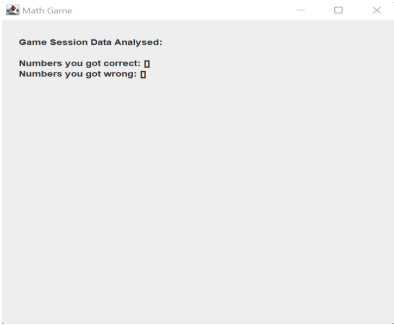top of page
Search

# Mini Math Game - Java Project Help

## Java project work:

Your task is to implement two classes named as App.java and MyFrame.java according to the below specifications:

• App.java will contain your main method.

• MyFrame.java will handle anything related to the Graphical User Interface (GUI)

For this task you will create a mini math game.

• App.java holds the main class to run the program:

• MyFrame.java is responsible for GUI. Anything that is related to GUI must declared here

Below you will find the description of the game with some screenshots. Your task is to

replicate the game logic as closely as possible.

Getting started:

start by defining the frame so you can get the Java GUI working.

• The size of the frame should be set to (500,500)

• The application title should be ‘Math Game’, as you can see (top window bar) in the screenshot below.Next, add the title and start button:

• A title message ‘Welcome to mini math game!’

• A button with text ‘Start’Once the user has clicked on the ‘Start’ button, the start menu item should disappear and

the game begin. The game should behave and display as shown below.

The game:

Let’s start with the logic of the game – make sure you understand this. In this game, the

computer will generate a random number (going forward, this will be referred to as the

‘Puzzle Number’) and the goal for the user is to select two numbers that add together to

make the Puzzle Number.

Example:

The following image displays the text ‘Select two numbers that add up to 3’, so 3 is the

Puzzle Number (a random number generated by the computer) and the user will need to

select two numbers that sum to 3. For 3, the answer is 1 and 2, so the user should select

buttons 1 and 2 or 2 and 1.

Once the user has made their selections, the program will check if they sum to the Puzzle

Number.• If the user selects the correct numbers, the program will increase the user ‘Win’ number.

• If the user selects the wrong numbers, the program will increase the ‘Lose’ number

After the program has checked the user answer and updated the result it will generate a new puzzle Number and display it to the user so the user can continue playing the game. This game can go on forever..

Important constraints:

• You need to pay attention to the random number. Be careful that you take into account the game logic – the user can only select two numbers between 1 and 9.

• Any new Puzzle Number must be different from the one generated immediately before. For example, if 3 was the Puzzle Number, the next Puzzle Number cannot be 3; however, if 3 was the Puzzle Number, and the next Puzzle Number was 4, the one after that could be 3 (but not 4).

• The user always must select two numbers; one number cannot be accepted as an answer.

Analysis of game data:Once the user clicks on the “End Game Button”, the game session data analysis should be displayed as follows.The order should follow the image above exactly. First give the title: “Game Session Data

Analysed:”. Leave a blank line and then display the analysed data:

• Numbers you got correct: show the Puzzle Numbers the user answered correctly, in order of play.

• Numbers you got wrong: show the Puzzle Numbers the user answered incorrectly, in order of play.

• Percentage of win for numbers greater than or equal to 10: display percentage (you need to figure out the logic to calculate the percentage).

• Percentage of win for numbers less than 10: display percentage (you need to figure out the logic to calculate the percentage).

The UI for both percentages should be dynamic as there might be cases where it is not

possible to calculate the percentage of win. In those cases, do not display the text for

percentage of win.

Example 1:

The user has played the game for 2 rounds and then ended the game. In these 2 rounds

they did not get any Puzzle Number greater than or equal to 10, so it is not possible to

calculate the percentage of win for number less 10, and the related text is not displayedExample 2:

The user has started the game but ended it before playing. It is not possible to calculate the percentage of win for numbers less 10 or greater than or equal to 10, so the related text is not displayed. However, while no Puzzle Numbers were generated, the display shows an empty array “[]” for both ‘numbers correct’ and ‘numbers wrong’Example 3:

The user plays the game for a long time, and when displaying the data, the text might go

over the application size (500x500). You need to figure out a way to adapt dynamically to

wrap the text and data elements so that they display within the screen.Improving the application UI. Here you need to use Java features to improve the overall look of the application. For example, you could display the game buttons differently, as below.### CodersArts provide :

• Java assignment Help

• Help in Java development Projects

• Mentorship from Experts Live 1:1 session

• Course and Project completions

• CourseWork help

See All
bottom of page